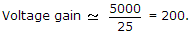# Electronics and Communication Engineering - Analog Electronics

16.

The inverting op-amp shown in the figure has an open loop gain to 100. The closed loop given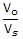is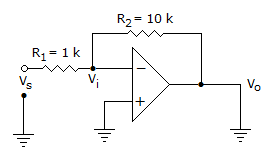A. -8 B. -9 C. -10 D. -11

Explanation:

Applying KCL inverting node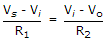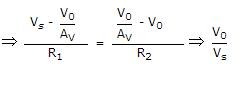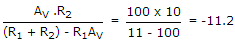.

17.

In a voltage regulated power supply the zener operates in the breakdown region when (Vin is input voltage and Vz is zener breakdown voltage)

 A. Vin < Vz B. Vin > Vz C. Vin = Vz D. both (a) or (b)

Explanation:

Zener breakdown when input voltage is more than zener breakdown voltage.

18.

The parameter h11 for CB circuit is higher than that for CE circuit.

 A. True B. False

Explanation:

It is lower.

19.

Figure shows a self bias circuit for FET amplifier, ID = 4 mA. Then IS =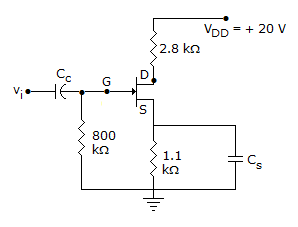A. 4 mA B. 2 mA C. 0.4 mA D. 0.2 mA

Explanation:

Since gate current is negligible IS = ID = 4 mA.

20.

In the CE equivalent circuit of figure, the voltage gain is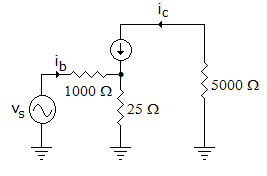A. 200 B. 40 C. 5 D. 1000